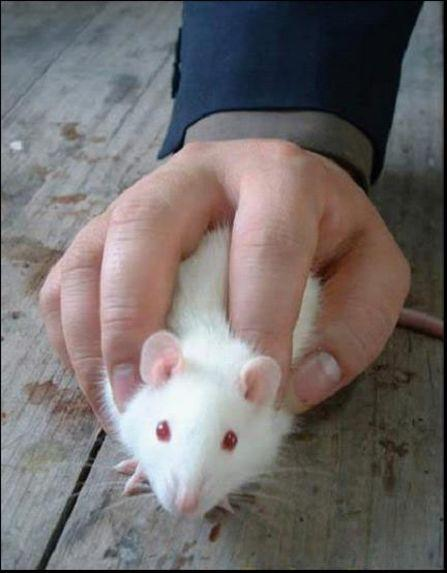windows 下用 C# 编程实现 键鼠模拟 ，这个是驱动级的，不会被游戏检测出来，可以干很多事情，你懂的。

## DD_btn(参数)

4 =右键按下 ，8 =右键放开
16 =中键按下 ，32 =中键放开
64 =4键按下 ，128 =4键放开
256 =5键按下 ，512 =5键放开

## DD_mov(参数x,参数y)

int x = 1920/2 ; int y = 1080/2;
DD_mov(x,y) ;

## DD_key(参数1，参数2)

`DD_key(300, 1);`
`DD_key(300, 2);`

## DD_str(参数)微信